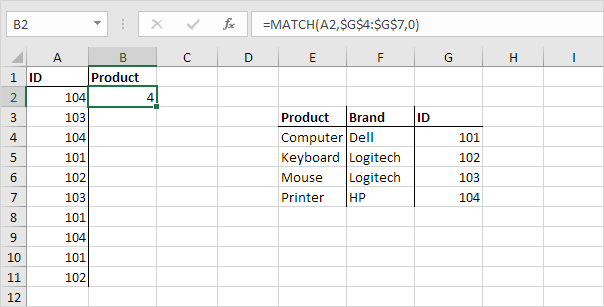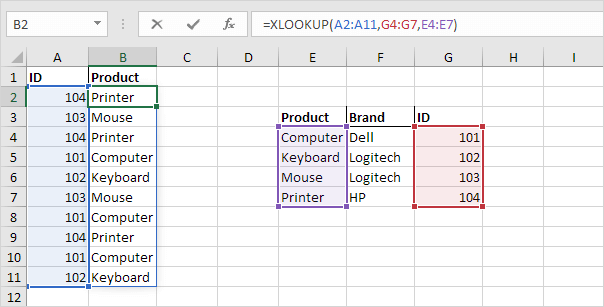# Left Lookup

The VLOOKUP function only looks to the right. To look up a value in any column and return the corresponding value to the left, simply use INDEX and MATCH.

1. The MATCH function returns the position of a value in a given range.Explanation: 104 found at position 4 in the range \$G\$4:\$G\$7.

2. Use this result and the INDEX function to return the 4th value in the range \$E\$4:\$E\$7.3. Drag the formula in cell B2 down to cell B11.Note: when we drag this formula down, the absolute references (\$E\$4:\$E\$7 and \$G\$4:\$G\$7) stay the same, while the relative reference (A2) changes to A3, A4, A5, etc.

4. If you have Excel 365 or Excel 2021, simply use the XLOOKUP function to perform a left lookup.